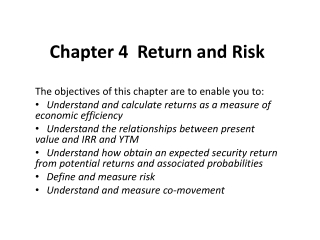DownloadDownload PresentationChapter 4 Return and Risk

# Chapter 4 Return and Risk

Télécharger la présentation## Chapter 4 Return and Risk

- - - - - - - - - - - - - - - - - - - - - - - - - - - E N D - - - - - - - - - - - - - - - - - - - - - - - - - - -
##### Presentation Transcript

1. Chapter 4 Return and Risk The objectives of this chapter are to enable you to: Understand and calculate returns as a measure of economic efficiency Understand the relationships between present value and IRR and YTM Understand how obtain an expected security return from potential returns and associated probabilities Define and measure risk Understand and measure co-movement

2. 4.A. INTRODUCTION • The purpose of measuring investment returns is simply to determine the economic efficiency of an investment. • An investment's return will express the profits generated by an initial cash outlay relative to the amount of that outlay.

3. 4.B. RETURN ON INVESTMENT: ARITHMETIC MEAN

4. Fund Performance

5. 4.C. RETURN MEASUREMENT: GEOMETRIC MEAN

6. Fund Performance Fund AFund B DatetPtPt-1DIVtrtPtPt-1DIVtrt June 30 0 50 - 0 - 50 - 0 - July 31 1 55 50 0 .100 80 50 0 .60 Aug. 31 2 50 55 0 -.091 40 80 0 -.50 Sep. 30 3 54 50 0 .080 60 40 0 .50 Oct. 31 4 47 54 2 -.092 30 60 0 -.50 Nov. 30 5 51 47 0 .081 45 30 0 .50

7. Fund Returns • Compare the prices and returns of two funds. • Recall that the arithmetic mean return on investment for Fund A is .0156. The arithmetic mean return on investment on Fund B is .12.

8. 4.D. INTERNAL RATE OF RETURN Consider a stock whose purchase price three years ago was \$100. This stock paid a dividend of \$10 in each of the three years and was sold for \$130. Its arithmetic mean annual return is 20%

9. IRR Strengths and Weaknesses • The primary advantage of the internal rate of return over return on investment is that it accounts for the timeliness of all cash flows. However, IRR does have three major weaknesses: 1. As we have seen, IRR takes longer to calculate than does ROI. 2. Sometimes an investment will generate multiple rates of return; that is, more than one (r) value will equate NPV with zero. This will occur when that investment has associated with it more than one negative cash flow. When multiple rates are generated, there is often no method to determine which is the true IRR. 3. The internal rate of return is based on the assumption that cash flows received prior to the expiration of the investment will be re-invested at the internal rate of return.

10. 4.E. BOND YIELDS The yield to maturity (y) of the 4-year \$1000 corporate bond above is 6% if it were purchased for \$1,000; the yield to maturity would be 12.7% if the purchase price were \$800.

11. 4.F. INTRODUCTION TO RISK • What is risk?

12. 4.G. EXPECTED RETURN Consider an economy with 3 potential states of nature in the next year If the economy performs well, state 1 is realized and the stock earns 25%. If the economy performs only satisfactorily, state 2is realized and the stock earns 10%. If the economy performs poorly, state 3is realized and the stock earns ‑10%. Assume that there is a 20% for state 1 will occur, a 50% chance for state 2 and a 30% for state 3. The expected return on the stock will be 7%: E[RA]= (.25 ×.20) + (.10 × .50) + (‑.10 × .30) = .07

13. 4.H: VARIANCE AND STANDARD DEVIATION The variance of stock A returns presented in Section 4.G is .0156: σ2= (.25‑.07)2 ×.2 + (.10‑.07)2 ×.5 + (‑.10‑.07)2 ×.3 = .0156 The standard deviation of returns on our stock is 12.49%.

14. 4.I: HISTORICAL VARIANCE AND STANDARD DEVIATION • Historical stock return variances (standard deviations) can be reasonable indicators of future variances (standard deviations). Table 4.4: Historical Variance and Standard Deviation of Returns of Stock D _ _ _ tRtRt - RD(Rt - RD)2(Rt - RD)2 1/n 1 .10 -.06 .0036 .00072 2 .15 -.01 .0001 .00002 3 .20 .04 .0016 .00032 4 .10 -.06 .0036 .00072 5.25 .09 .0081 .00162 Rd=.16 σ2 =.00340 σd =.05831

15. 4.J. COVARIANCE Table 4.5: Covariance between Returns on Stocks A and B iRaiRbiPi Rai-E[Ra]Rbi-E[Rb](Rai-E[Ra])(Rbi-E[Rb])Pi 1 .25 .45 .20 .18 .38 .01368 2 .10 .05 .50 .03 -.02 -.00030 3 -.10 -.15 .30 -.17 -.22 .01122 COV(A,B) = .0246 cov(A,B) = {(.25 ‑ .07) × (.45 ‑ .07) × .20} + {(.10 ‑ .07) × (.05 ‑ .07) × .50} + {(‑.10 ‑.07) × (‑.15 ‑.07) × .30} = {.01368} + {-.0003} + {.01122} = .0246.

16. Historical Covariance Covariance measures co-movement tendency. Table 4.6: Historical Covariance between Stocks D and E _ _ _ _ tRdtRet(Rdt-Rd)(Ret-Re)(Rdt-Rd)(Ret-Re)1/n 1 .10 .15 -.06 -.05 .00060 2 .15 .18 -.01 -.02 .00004 3 .20 .25 .04 .05 .00040 4 .10 .20 -.06 0 0 5 .25 .22 .09 .02 .00036 _ _ RD=.16 .20=RE COV(D,E) = .00140

17. 4.K: COEFFICIENT OF CORRELATION Correlation coefficient is a measure of co-movement standardized to fall between -1 and 1. COV(k,j) = σkσjρk,j

18. 4.L: THE MARKET PORTFOLIO • Dow Jones Industrial Average • Standard and Poor's 500 (S&P 500) • Shanghai SE Composite Index